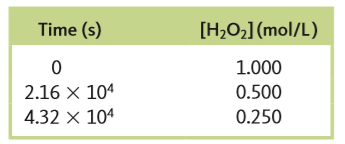# Problem: At 40°C, H2O2(aq) will decompose according to the following reaction:2H2O2 (aq) → 2H2O (l) + O2 (g)The following data were collected for the concentration of H 2O2 at various times.a. Calculate the average rate of decomposition of H 2O2 between 0 and 2.16 x 104 s. Use this rate to calculate the average rate of production of O2(g) over the same time period.

###### FREE Expert Solution
81% (240 ratings)
###### FREE Expert Solution

We are asked to calculate the average rate of decomposition of H2O2 and O2 in the given reaction:

2 H2O2 (aq) → 2 H2O (l)   +   O(g)

We’re given the following data:

 Time (s) [H2O2] (mol/L) 0 1.000 2.16 × 104 0.500 4.32 × 104 0.250

81% (240 ratings)###### Problem Details

At 40°C, H2O2(aq) will decompose according to the following reaction:

2H2O(aq) → 2H2O (l) + O2 (g)

The following data were collected for the concentration of H 2O2 at various times.a. Calculate the average rate of decomposition of H 2O2 between 0 and 2.16 x 104 s. Use this rate to calculate the average rate of production of O2(g) over the same time period.

Frequently Asked Questions

What scientific concept do you need to know in order to solve this problem?

Our tutors have indicated that to solve this problem you will need to apply the Average Rate of Reaction concept. You can view video lessons to learn Average Rate of Reaction. Or if you need more Average Rate of Reaction practice, you can also practice Average Rate of Reaction practice problems.

What is the difficulty of this problem?

Our tutors rated the difficulty ofAt 40°C, H2O2(aq) will decompose according to the following ...as medium difficulty.

How long does this problem take to solve?

Our expert Chemistry tutor, Sabrina took 4 minutes and 21 seconds to solve this problem. You can follow their steps in the video explanation above.

What professor is this problem relevant for?

Based on our data, we think this problem is relevant for Professor Tezcan's class at UCSD.

What textbook is this problem found in?

Our data indicates that this problem or a close variation was asked in Chemistry: An Atoms First Approach - Zumdahl Atoms 1st 2nd Edition. You can also practice Chemistry: An Atoms First Approach - Zumdahl Atoms 1st 2nd Edition practice problems.